# NCERT solutions for Mathematics Exemplar Class 10 chapter 11 - Area Related To Circles [Latest edition]

#### Chapters## Chapter 11: Area Related To Circles

Exercise 11.1Exercise 11.2Exercise 11.3Exercise 11.4
Exercise 11.1 [Pages 120 - 121]

### NCERT solutions for Mathematics Exemplar Class 10 Chapter 11 Area Related To Circles Exercise 11.1 [Pages 120 - 121]

#### Choose the correct alternative:

Exercise 11.1 | Q 1 | Page 120

If the sum of the areas of two circles with radii R1 and R2 is equal to the area of a circle of radius R, then ______.

• R1 + R2 = R

• "R"_1^2 + "R"_2^2 < "R"^2

• R1 + R2 < R

• "R"_1^2 + "R"_2^2 < "R"^2

Exercise 11.1 | Q 2 | Page 120

If the sum of the circumferences of two circles with radii R1 and R2 is equal to the circumference of a circle of radius R, then ______.

• R1 + R2 = R

• R1 + R2 > R

• R1 + R2 < R

• Nothing definite can be said about the relation among R1, R2 and R.

Exercise 11.1 | Q 3 | Page 121

If the circumference of a circle and the perimeter of a square are equal, then ______.

• Area of the circle = Area of the square

• Area of the circle  < Area of the  square

• Area of the circle > Area of the square

• Nothing definite can be said about the relation between the areas of the circle and square.

Exercise 11.1 | Q 4 | Page 121

Area of the largest triangle that can be inscribed in a semi-circle of radius r units is ______.

• rsq.units

• 1/2 rsq.units

•  2 rsq.units

• sqrt(2) rsq.units

Exercise 11.1 | Q 5 | Page 121

If the perimeter of a circle is equal to that of a square, then the ratio of their areas is ______.

•  13 : 22

• 14 : 11

•  22 : 13

• 11 : 14

Exercise 11.1 | Q 6 | Page 121

It is proposed to build a single circular park equal in area to the sum of areas of two circular parks of diameters 16 m and 12 m in a locality. The radius of the new park would be ______.

• 10 m

• 15 m

• 20 m

• 24 m

Exercise 11.1 | Q 7 | Page 121

The area of the circle that can be inscribed in a square of side 6 cm, is ______.

• 36π cm2

• 18π cm2

• 12π cm2

• 9π cm2

Exercise 11.1 | Q 8 | Page 121

The area of the square that can be inscribed in a circle of radius 8 cm is ______.

• 256 cm2

• 128 cm2

• 64sqrt(2) cm2

• 64 cm2

Exercise 11.1 | Q 9 | Page 121

The radius of a circle whose circumference is equal to the sum of the circumferences of the two circles of diameters 36 cm and 20 cm is ______.

• 56 cm

• 42 cm

• 28 cm

• 16 cm

Exercise 11.1 | Q 10 | Page 121

The diameter of a circle whose area is equal to the sum of the areas of the two circles of radii 24 cm and 7 cm is ______.

• 31 cm

• 25 cm

• 62 cm

• 50 cm

Exercise 11.2 [Pages 122 - 123]

### NCERT solutions for Mathematics Exemplar Class 10 Chapter 11 Area Related To Circles Exercise 11.2 [Pages 122 - 123]

#### State whether the following statement is True or False:

Exercise 11.2 | Q 1 | Page 122

Is the area of the circle inscribed in a square of side a cm, πa2 cm2? Give reasons for your answer.

• True

• False

Exercise 11.2 | Q 2 | Page 122

Will it be true to say that the perimeter of a square circumscribing a circle of radius a cm is 8a cm? Give reasons for your answer.

• True

• False

Exercise 11.2 | Q 3 | Page 122

In figure, a square is inscribed in a circle of diameter d and another square is circumscribing the circle. Is the area of the outer square four times the area of the inner square? Give reasons for your answer.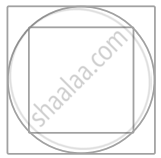• True

• False

Exercise 11.2 | Q 4 | Page 123

Is it true to say that area of a segment of a circle is less than the area of its corresponding sector? Why?

• True

• False

Exercise 11.2 | Q 5 | Page 123

Is it true that the distance travelled by a circular wheel of diameter d cm in one revolution is 2 π d cm? Why?

• True

• False

Exercise 11.2 | Q 6 | Page 123

In covering a distance s metres, a circular wheel of radius r metres makes s/(2 pir) revolutions. Is this statement true? Why?

• True

• False

Exercise 11.2 | Q 7 | Page 123

The numerical value of the area of a circle is greater than the numerical value of its circumference. Is this statement true? Why?

• True

• False

Exercise 11.2 | Q 8 | Page 123

If the length of an arc of a circle of radius r is equal to that of an arc of a circle of radius 2r, then the angle of the corresponding sector of the first circle is double the angle of the corresponding sector of the other circle. Is this statement false? Why?

• True

• False

Exercise 11.2 | Q 9 | Page 123

The areas of two sectors of two different circles with equal corresponding arc lengths are equal. Is this statement true? Why?

• True

• False

Exercise 11.2 | Q 10 | Page 123

The areas of two sectors of two different circles are equal. Is it necessary that their corresponding arc lengths are equal? Why?

• True

• False

Exercise 11.2 | Q 11 | Page 123

Is the area of the largest circle that can be drawn inside a rectangle of length a cm and breadth b cm (a > b) is πb2 cm2? Why?

• True

• False

Exercise 11.2 | Q 12 | Page 123

Circumferences of two circles are equal. Is it necessary that their areas be equal? Why?

• True

• False

Exercise 11.2 | Q 13 | Page 123

Areas of two circles are equal. Is it necessary that their circumferences are equal? Why?

• True

• False

Exercise 11.2 | Q 14 | Page 123

Is it true to say that area of a square inscribed in a circle of diameter p cm is p2 cm2? Why?

• True

• False

Exercise 11.3 [Pages 125 - 128]

### NCERT solutions for Mathematics Exemplar Class 10 Chapter 11 Area Related To Circles Exercise 11.3 [Pages 125 - 128]

#### Unless stated otherwise, use π = 22/7

Exercise 11.3 | Q 1 | Page 125

Find the radius of a circle whose circumference is equal to the sum of the circumferences of two circles of radii 15 cm and 18 cm.

Exercise 11.3 | Q 2 | Page 125

In figure, a square of diagonal 8 cm is inscribed in a circle. Find the area of the shaded region.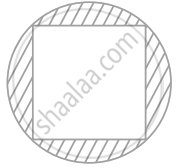Exercise 11.3 | Q 3 | Page 126

Find the area of a sector of a circle of radius 28 cm and central angle 45°.

Exercise 11.3 | Q 4 | Page 126

The wheel of a motor cycle is of radius 35 cm . How many revolutions per minute must the wheel make so as to keep a speed of 66 km / hr ?

Exercise 11.3 | Q 5 | Page 126

A cow is tied with a rope of length 14 m at the corner of a rectangular  field of dimensions 20 m xx 16 m  find the area of the field in which the cow can graze .

Exercise 11.3 | Q 6 | Page 126

Find the area of the flower bed (with semi-circular ends) shown in figure.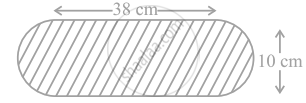Exercise 11.3 | Q 7 | Page 126

In figure, AB is a diameter of the circle, AC = 6 cm and BC = 8 cm. Find the area of the shaded region (Use π = 3.14).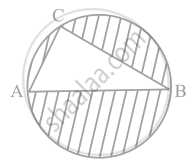Exercise 11.3 | Q 8 | Page 126

Find the area of the shaded field shown in figure.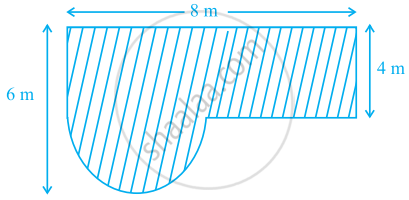Exercise 11.3 | Q 9 | Page 127

Find the area of the shaded region in figure.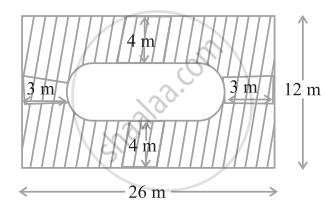Exercise 11.3 | Q 10 | Page 127

Find the area of the minor segment of a circle of radius 14 cm, when the angle of the corresponding sector is 60°.

Exercise 11.3 | Q 11 | Page 127

Find the area of the shaded region in figure, where arcs drawn with centres A, B, C and D intersect in pairs at mid-points P, Q, R and S of the sides AB, BC, CD and DA, respectively of a square ABCD (Use π = 3.14).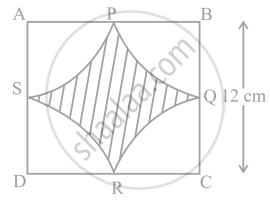Exercise 11.3 | Q 12 | Page 127

In figure, arcs are drawn by taking vertices A, B and C of an equilateral triangle of side 10 cm. to intersect the sides BC, CA and AB at their respective mid-points D, E and F. Find the area of the shaded region (Use π = 3.14).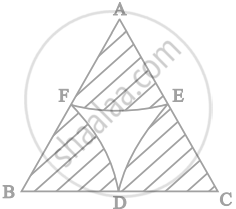Exercise 11.3 | Q 13 | Page 128

In figure, arcs have been drawn with radii 14 cm each and with centres P, Q and R. Find the area of the shaded region.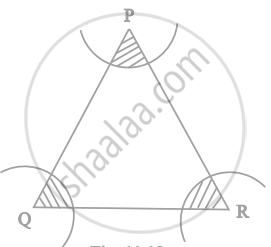Exercise 11.3 | Q 14 | Page 128

A circular park is surrounded by a road 21 m wide. If the radius of the park is 105 m, find the area of the road.

Exercise 11.3 | Q 15 | Page 128

In figure, arcs have been drawn of radius 21 cm each with vertices A, B, C and D of quadrilateral ABCD as centres. Find the area of the shaded region.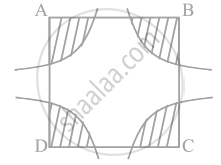Exercise 11.3 | Q 16 | Page 128

A piece of wire 20 cm long is bent into the form of an arc of a circle subtending an angle of 60° at its centre. Find the radius of the circle.

Exercise 11.4 [Pages 132 - 135]

### NCERT solutions for Mathematics Exemplar Class 10 Chapter 11 Area Related To Circles Exercise 11.4 [Pages 132 - 135]

Exercise 11.4 | Q 1 | Page 132

The area of a circular playground is 22176 m2. Find the cost of fencing this ground at the rate of Rs 50 per metre.

Exercise 11.4 | Q 2 | Page 132

The diameters of front and rear wheels of a tractor are 80 cm and 2 m respectively. Find the number of revolutions that rear wheel will make in covering a distance in which the front wheel makes 1400 revolutions.

Exercise 11.4 | Q 3 | Page 132

Sides of a triangular field are 15 m, 16 m and 17 m. With the three corners of the field a cow, a buffalo and a horse are tied separately with ropes of length 7 m each to graze in the field. Find the area of the field which cannot be grazed by the three animals.

Exercise 11.4 | Q 4 | Page 133

Find the area of the segment of a circle of radius 12 cm whose corresponding sector has a central angle of 60° (Use π = 3.14).

Exercise 11.4 | Q 5 | Page 133

A circular pond is 17.5 m is of diameter. It is surrounded by a 2 m wide path. Find the cost of constructing the path at the rate of Rs 25 per m2

Exercise 11.4 | Q 6 | Page 133

In figure, ABCD is a trapezium with AB || DC, AB = 18 cm, DC = 32 cm and distance between AB and DC = 14 cm. If arcs of equal radii 7 cm with centres A, B, C and D have been drawn, then find the area of the shaded region of the figure.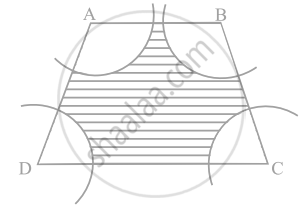Exercise 11.4 | Q 7 | Page 133

Three circles each of radius 3.5 cm are drawn in such a way that each of them touches the other two. Find the area enclosed between these circles.

Exercise 11.4 | Q 8 | Page 133

Find the area of the sector of a circle of radius 5 cm, if the corresponding arc length is 3.5 cm.

Exercise 11.4 | Q 9 | Page 133

Four circular cardboard pieces of radii 7 cm are placed on a paper in such a way that each piece touches other two pieces. Find the area of the portion enclosed between these pieces.

Exercise 11.4 | Q 10 | Page 133

On a square cardboard sheet of area 784 cm2, four congruent circular plates of maximum size are placed such that each circular plate touches the other two plates and each side of the square sheet is tangent to two circular plates. Find the area of the square sheet not covered by the circular plates.

Exercise 11.4 | Q 11 | Page 133

Floor of a room is of dimensions 5 m × 4 m and it is covered with circular tiles of diameters 50 cm each as shown in figure. Find the area of floor that remains uncovered with tiles. (Use π = 3.14)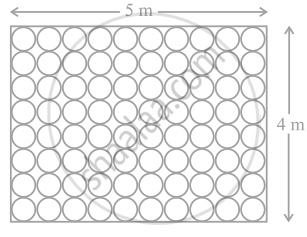Exercise 11.4 | Q 12 | Page 134

All the vertices of a rhombus lie on a circle. Find the area of the rhombus, if the area of the circle is 1256 cm2. (Use π = 3.14)

Exercise 11.4 | Q 13 | Page 134

An archery target has three regions formed by three concentric circles as shown in figure. If the diameters of the concentric circles are in the ratios 1 : 2 : 3 , then find the ratio of the areas of three regions.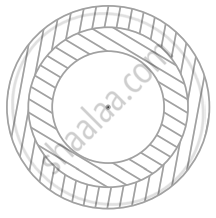Exercise 11.4 | Q 14 | Page 134

The length of the minute hand of a clock is 5 cm. Find the area swept by the minute hand during the time period 6:05 am and 6:40 am.

Exercise 11.4 | Q 15 | Page 134

Area of a sector of central angle 200° of a circle is 770 cm2. Find the length of the corresponding arc of this sector.

Exercise 11.4 | Q 16 | Page 135

The central angles of two sectors of circles of radii 7 cm and 21 cm are respectively 120° and 40°. Find the areas of the two sectors as well as the lengths of the corresponding arcs. What do you observe?

Exercise 11.4 | Q 17 | Page 135

Find the area of the shaded region given in figure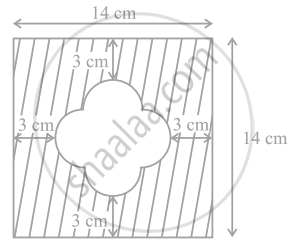Exercise 11.4 | Q 18 | Page 135

Find the number of revolutions made by a circular wheel of area 1.54 m2 in rolling a distance of 176 m.

Exercise 11.4 | Q 19 | Page 135

Find the difference of the areas of two segments of a circle formed by a chord of length 5 cm subtending an angle of 90° at the centre.

Exercise 11.4 | Q 20 | Page 135

Find the difference of the areas of a sector of angle 120° and its corresponding major sector of a circle of radius 21 cm.

## Chapter 11: Area Related To Circles

Exercise 11.1Exercise 11.2Exercise 11.3Exercise 11.4## NCERT solutions for Mathematics Exemplar Class 10 chapter 11 - Area Related To Circles

NCERT solutions for Mathematics Exemplar Class 10 chapter 11 (Area Related To Circles) include all questions with solution and detail explanation. This will clear students doubts about any question and improve application skills while preparing for board exams. The detailed, step-by-step solutions will help you understand the concepts better and clear your confusions, if any. Shaalaa.com has the CBSE Mathematics Exemplar Class 10 solutions in a manner that help students grasp basic concepts better and faster.

Further, we at Shaalaa.com provide such solutions so that students can prepare for written exams. NCERT textbook solutions can be a core help for self-study and acts as a perfect self-help guidance for students.

Concepts covered in Mathematics Exemplar Class 10 chapter 11 Area Related To Circles are Areas of Sector and Segment of a Circle, Area of Circle, Areas of Combinations of Plane Figures, Circumference of a Circle, Circumference of a Circle.

Using NCERT Class 10 solutions Area Related To Circles exercise by students are an easy way to prepare for the exams, as they involve solutions arranged chapter-wise also page wise. The questions involved in NCERT Solutions are important questions that can be asked in the final exam. Maximum students of CBSE Class 10 prefer NCERT Textbook Solutions to score more in exam.

Get the free view of chapter 11 Area Related To Circles Class 10 extra questions for Mathematics Exemplar Class 10 and can use Shaalaa.com to keep it handy for your exam preparation